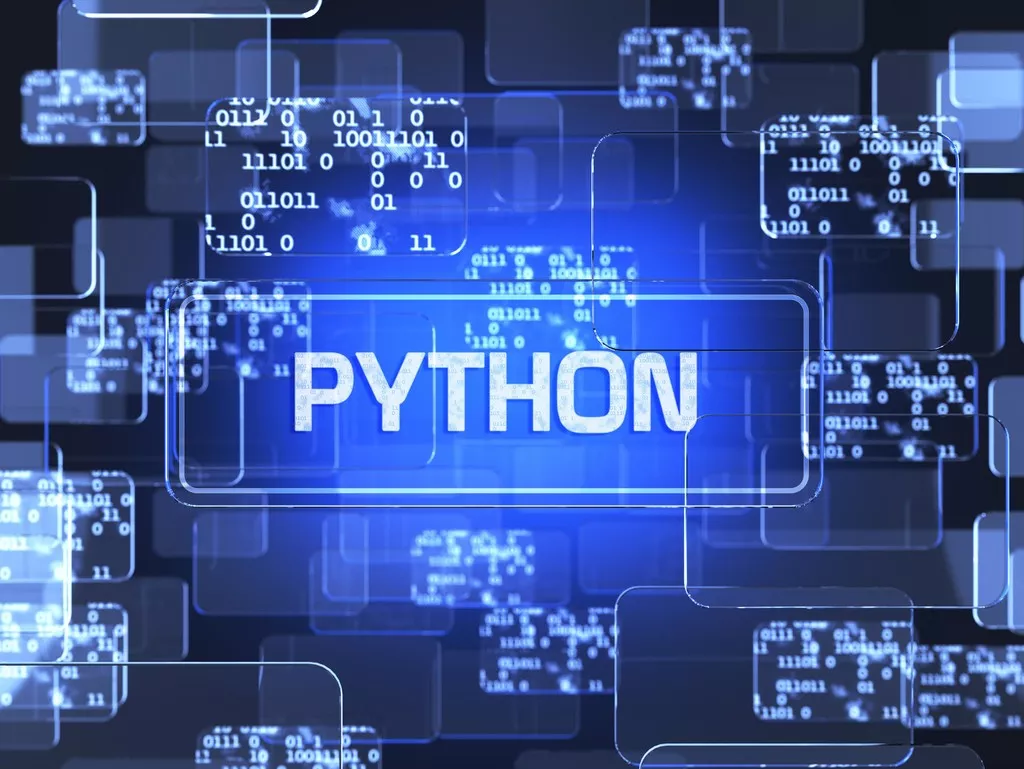# 如何让Python代码加速运行？

Python 是一种脚本语言，相比 C/C++ 这样的编译语言，在效率和性能方面存在一些不足。但是，有很多时候，Python 的效率并没有想象中的那么夸张。本文对一些 Python…

Python 是一种脚本语言，相比 C/C++ 这样的编译语言，在效率和性能方面存在一些不足。但是，有很多时候，Python 的效率并没有想象中的那么夸张。本文对一些 Python 代码加速运行的技巧进行整理。### 1. 避免全局变量

``````# 不推荐写法。代码耗时：26.8秒
import math

size = 10000
for x in range(size):
for y in range(size):
z = math.sqrt(x) + math.sqrt(y)
``````

``````# 推荐写法。代码耗时：20.6秒
import math

def main():  # 定义到函数中，以减少全部变量使用
size = 10000
for x in range(size):
for y in range(size):
z = math.sqrt(x) + math.sqrt(y)

main()
``````

### 2. 避免.（属性访问操作符）

#### 2.1 避免模块和函数属性访问

``````# 不推荐写法。代码耗时：14.5秒
import math

def computeSqrt(size: int):
result = []
for i in range(size):
result.append(math.sqrt(i))
return result

def main():
size = 10000
for _ in range(size):
result = computeSqrt(size)

main()
``````

``````# 第一次优化写法。代码耗时：10.9秒
from math import sqrt

def computeSqrt(size: int):
result = []
for i in range(size):
result.append(sqrt(i))  # 避免math.sqrt的使用
return result

def main():
size = 10000
for _ in range(size):
result = computeSqrt(size)

main()
``````

``````# 第二次优化写法。代码耗时：9.9秒
import math

def computeSqrt(size: int):
result = []
sqrt = math.sqrt  # 赋值给局部变量
for i in range(size):
result.append(sqrt(i))  # 避免math.sqrt的使用
return result

def main():
size = 10000
for _ in range(size):
result = computeSqrt(size)

main()
``````

``````# 推荐写法。代码耗时：7.9秒
import math

def computeSqrt(size: int):
result = []
append = result.append
sqrt = math.sqrt    # 赋值给局部变量
for i in range(size):
append(sqrt(i))  # 避免 result.append 和 math.sqrt 的使用
return result

def main():
size = 10000
for _ in range(size):
result = computeSqrt(size)

main()
``````

#### 2.2 避免类内属性访问

``````# 不推荐写法。代码耗时：10.4秒
import math
from typing import List

class DemoClass:
def __init__(self, value: int):
self._value = value

def computeSqrt(self, size: int) -> List[float]:
result = []
append = result.append
sqrt = math.sqrt
for _ in range(size):
append(sqrt(self._value))
return result

def main():
size = 10000
for _ in range(size):
demo_instance = DemoClass(size)
result = demo_instance.computeSqrt(size)

main()
``````

``````# 推荐写法。代码耗时：8.0秒
import math
from typing import List

class DemoClass:
def __init__(self, value: int):
self._value = value

def computeSqrt(self, size: int) -> List[float]:
result = []
append = result.append
sqrt = math.sqrt
value = self._value
for _ in range(size):
append(sqrt(value))  # 避免 self._value 的使用
return result

def main():
size = 10000
for _ in range(size):
demo_instance = DemoClass(size)
demo_instance.computeSqrt(size)

main()
``````

### 3. 避免不必要的抽象

``````# 不推荐写法，代码耗时：0.55秒
class DemoClass:
def __init__(self, value: int):
self.value = value

@property
def value(self) -> int:
return self._value

@value.setter
def value(self, x: int):
self._value = x

def main():
size = 1000000
for i in range(size):
demo_instance = DemoClass(size)
value = demo_instance.value
demo_instance.value = i

main()
``````

``````# 推荐写法，代码耗时：0.33秒
class DemoClass:
def __init__(self, value: int):
self.value = value  # 避免不必要的属性访问器

def main():
size = 1000000
for i in range(size):
demo_instance = DemoClass(size)
value = demo_instance.value
demo_instance.value = i

main()
``````

### 4. 避免数据复制

#### 4.1 避免无意义的数据复制

``````# 不推荐写法，代码耗时：6.5秒
def main():
size = 10000
for _ in range(size):
value = range(size)
value_list = [x for x in value]
square_list = [x * x for x in value_list]

main()
``````

``````# 推荐写法，代码耗时：4.8秒
def main():
size = 10000
for _ in range(size):
value = range(size)
square_list = [x * x for x in value]  # 避免无意义的复制

main()
``````

#### 4.2 交换值时不使用中间变量

``````# 不推荐写法，代码耗时：0.07秒
def main():
size = 1000000
for _ in range(size):
a = 3
b = 5
temp = a
a = b
b = temp

main()
``````

``````# 推荐写法，代码耗时：0.06秒
def main():
size = 1000000
for _ in range(size):
a = 3
b = 5
a, b = b, a  # 不借助中间变量

main()
``````

#### 4.3 字符串拼接用join而不是+

``````# 不推荐写法，代码耗时：2.6秒
import string
from typing import List

def concatString(string_list: List[str]) -> str:
result = ''
for str_i in string_list:
result += str_i
return result

def main():
string_list = list(string.ascii_letters * 100)
for _ in range(10000):
result = concatString(string_list)

main()
``````

``````# 推荐写法，代码耗时：0.3秒
import string
from typing import List

def concatString(string_list: List[str]) -> str:
return ''.join(string_list)  # 使用 join 而不是 +

def main():
string_list = list(string.ascii_letters * 100)
for _ in range(10000):
result = concatString(string_list)

main()
``````

### 5. 利用if条件的短路特性

``````# 不推荐写法，代码耗时：0.05秒
from typing import List

def concatString(string_list: List[str]) -> str:
abbreviations = {'cf.', 'e.g.', 'ex.', 'etc.', 'flg.', 'i.e.', 'Mr.', 'vs.'}
abbr_count = 0
result = ''
for str_i in string_list:
if str_i in abbreviations:
result += str_i
return result

def main():
for _ in range(10000):
string_list = ['Mr.', 'Hat', 'is', 'Chasing', 'the', 'black', 'cat', '.']
result = concatString(string_list)

main()
``````

if 条件的短路特性是指对if a and b这样的语句， 当a为False时将直接返回，不再计算b；对于if a or b这样的语句，当a为True时将直接返回，不再计算b。因此， 为了节约运行时间，对于or语句，应该将值为True可能性比较高的变量写在or前，而and应该推后。

``````# 推荐写法，代码耗时：0.03秒
from typing import List

def concatString(string_list: List[str]) -> str:
abbreviations = {'cf.', 'e.g.', 'ex.', 'etc.', 'flg.', 'i.e.', 'Mr.', 'vs.'}
abbr_count = 0
result = ''
for str_i in string_list:
if str_i[-1] == '.' and str_i in abbreviations:  # 利用 if 条件的短路特性
result += str_i
return result

def main():
for _ in range(10000):
string_list = ['Mr.', 'Hat', 'is', 'Chasing', 'the', 'black', 'cat', '.']
result = concatString(string_list)

main()
``````

### 6. 循环优化

#### 6.1 用for循环代替while循环

``````# 不推荐写法。代码耗时：6.7秒
def computeSum(size: int) -> int:
sum_ = 0
i = 0
while i < size:
sum_ += i
i += 1
return sum_

def main():
size = 10000
for _ in range(size):
sum_ = computeSum(size)

main()
``````

Python 的for循环比while循环快不少。

``````# 推荐写法。代码耗时：4.3秒
def computeSum(size: int) -> int:
sum_ = 0
for i in range(size):  # for 循环代替 while 循环
sum_ += i
return sum_

def main():
size = 10000
for _ in range(size):
sum_ = computeSum(size)

main()
``````

#### 6.2 使用隐式for循环代替显式for循环

``````# 推荐写法。代码耗时：1.7秒
def computeSum(size: int) -> int:
return sum(range(size))  # 隐式 for 循环代替显式 for 循环

def main():
size = 10000
for _ in range(size):
sum = computeSum(size)

main()
``````

#### 6.3 减少内层for循环的计算

``````# 不推荐写法。代码耗时：12.8秒
import math

def main():
size = 10000
sqrt = math.sqrt
for x in range(size):
for y in range(size):
z = sqrt(x) + sqrt(y)

main()
``````

``````# 推荐写法。代码耗时：7.0秒
import math

def main():
size = 10000
sqrt = math.sqrt
for x in range(size):
sqrt_x = sqrt(x)  # 减少内层 for 循环的计算
for y in range(size):
z = sqrt_x + sqrt(y)

main()
``````

### 为您推荐## Windows系统怎么保持远程桌面长时间链接不会自动断开呢?## Win11预览版 Builds 22572.100更新补丁KB5012817发布(附更新修复内容汇总)## Win11预览版全新标签式文件资源管理器上手体验：快速切换窗口，还可以滚动标签## 微软 Win11 22H2“太阳谷 2”重大版本将全面改造升级传统经典 UI